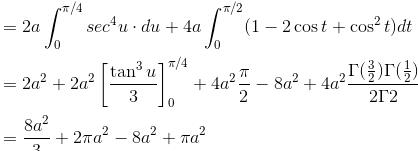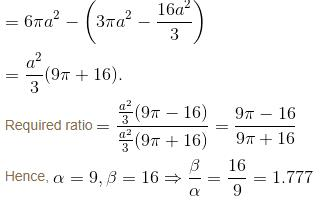Courses

# Integral Calculus NAT Level - 2

## 10 Questions MCQ Test Topic wise Tests for IIT JAM Physics | Integral Calculus NAT Level - 2

Description
This mock test of Integral Calculus NAT Level - 2 for Physics helps you for every Physics entrance exam. This contains 10 Multiple Choice Questions for Physics Integral Calculus NAT Level - 2 (mcq) to study with solutions a complete question bank. The solved questions answers in this Integral Calculus NAT Level - 2 quiz give you a good mix of easy questions and tough questions. Physics students definitely take this Integral Calculus NAT Level - 2 exercise for a better result in the exam. You can find other Integral Calculus NAT Level - 2 extra questions, long questions & short questions for Physics on EduRev as well by searching above.
*Answer can only contain numeric values
QUESTION: 1

### Show that the area common to the ellipses a2x2 + b2y2 = 1, b2x2 + a2y2 = 1. when 0 < a < b is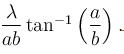Find the value of λ.

Solution: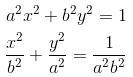This is a horizontal ellipse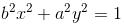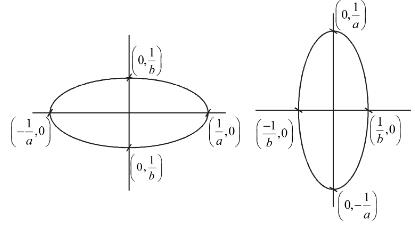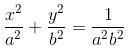This is a vertical ellipse
Total area = 4 x Area of OABC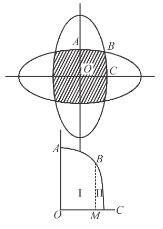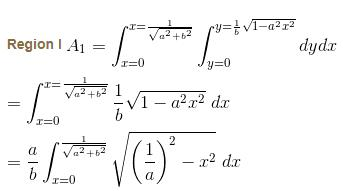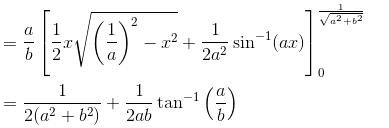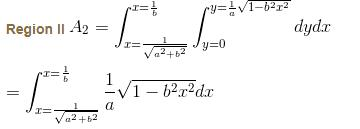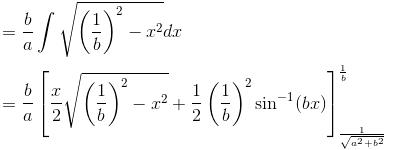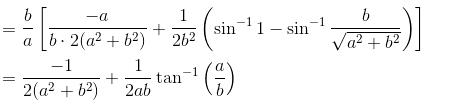Total area in first quadrant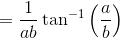Total area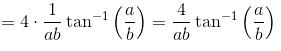Hence, value of λ = 4

*Answer can only contain numeric values
QUESTION: 2

### If the area shaded in the given figure is of the form λa2. Find the value of λ given the cardioids are r = a(1 + cosθ) and r = a(1 – cosθ) and a given circle r = a.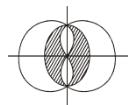Solution:

Let us first calculate the area included between two cardioids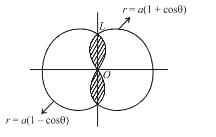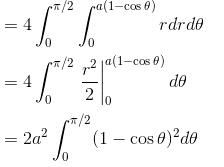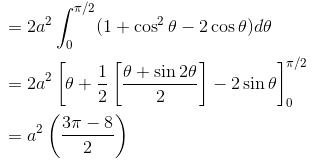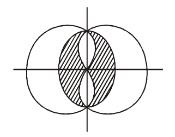Now, this shaded portion lies entirely inside the circle r = a with area πa2

Hence, required area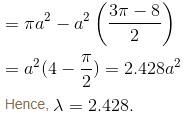*Answer can only contain numeric values
QUESTION: 3

### Transform the integral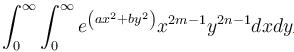to polar coordinates and if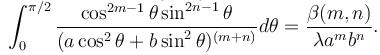Find the value of λ.

Solution:

Slope of this curve is equation of the curve x = a cos3t, y = b sin3t.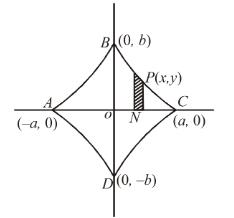Total Area = 4 × area OABO
This is of parametric form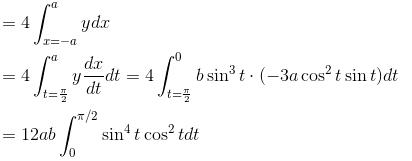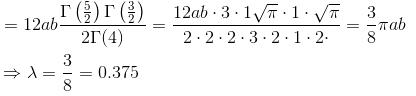*Answer can only contain numeric values
QUESTION: 4

Find the area of one loop of the curve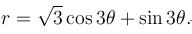If the area is of the form λπ.  Find the value of λ.

Solution:

The equation of the curve is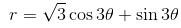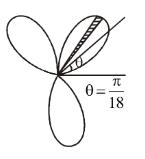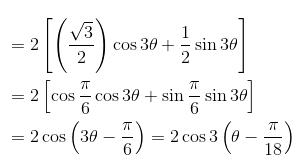Turn the initial line through an angle π/18 and putting θ as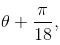the above equation reduces to r = 2 cos 3θ and the tracing of this curve is as in figure.

Also for r = 2 cos 3θ when r = 0 we get cos 3θ = 0 or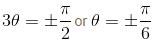and these is also symmetry about the initial line.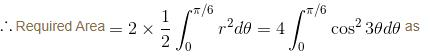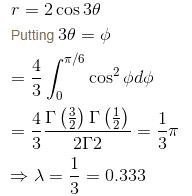*Answer can only contain numeric values
QUESTION: 5

If the whole area of the curve given by the equation x = a cos3 t,  y = b sin3 t or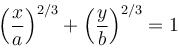is of the form λπab.  Find the value of λ.

Solution:

Slope of this curve is equation of the curve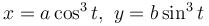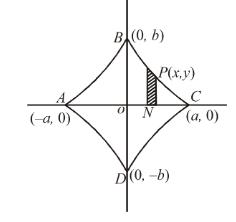Total Area = 4 × area OABO
This is of parametric form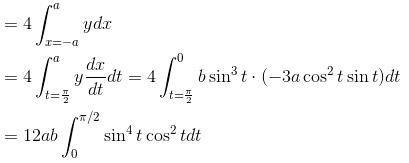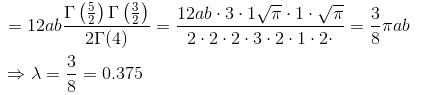*Answer can only contain numeric values
QUESTION: 6

If the area between the curve x(x2 + y2) = a(x2 – y2) and its asymptote is A1 and the area of the loop is A2 Then value of A1 + A2 = λ·a2. Find the value of λ.

Solution: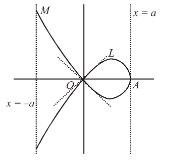The curve is symmetrical about x-axis. The loop is situated between lines x = 0 and x = a. The line x = a is asymptote of the curve,

We have,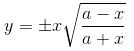For any point on arc OLA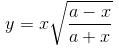For any point on arc OMB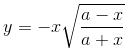Area between curve and its asymptotes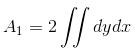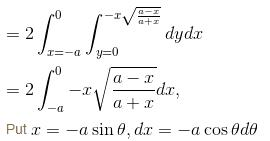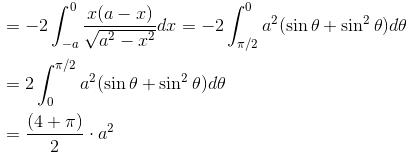Area of the loop is given as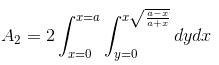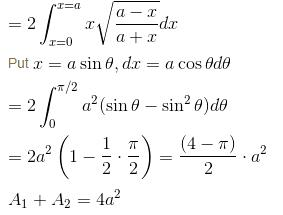Hence, λ = 4.

*Answer can only contain numeric values
QUESTION: 7

Find the area lying outside the circle r = 2acosθ and inside the cardioid r = a(1 + cosθ). This is of form λπa2. Find value of λ.

Solution:

Hence, the required area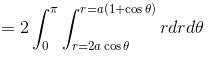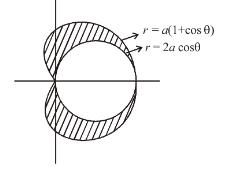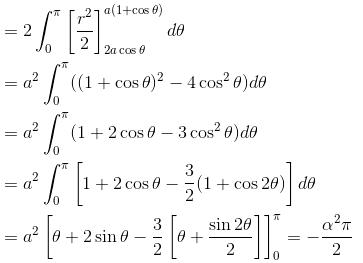Taking Mod sign we get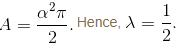*Answer can only contain numeric values
QUESTION: 8

Evaluate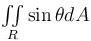where R is the region in the first quadrant that is outside the circle r = 2 and inside the cardioid r = 2(1 + cosθ).

Solution: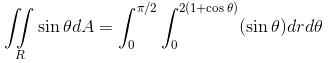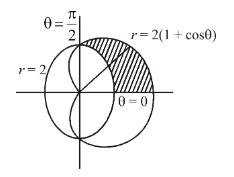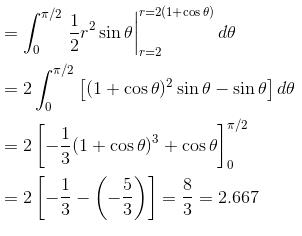*Answer can only contain numeric values
QUESTION: 9

The value of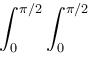sin x sin-1 (sin x sin y) dxdy is

Solution:

Let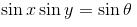Then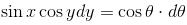keeping x constant when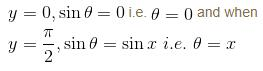Hence, θ varies from 0 to x.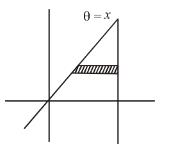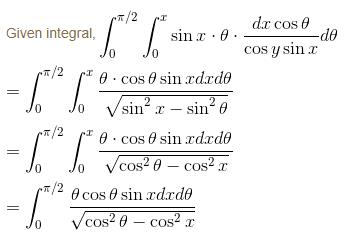Changing the order of integration with the help of figure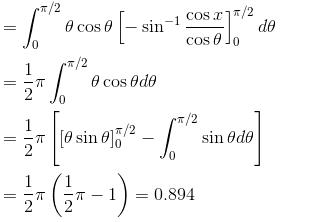*Answer can only contain numeric values
QUESTION: 10

If the ratio of the two parts into which the parabola 2a = r(1 + cosθ) divides the area of the cardiod r = 2a(1 + cosθ)  is of the form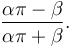Find the value of β/α.

Solution:

Solving the given equation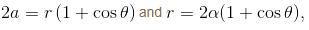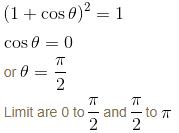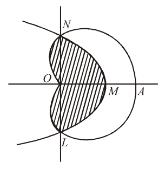Therefore shaded area = area OLMN
= 2 × area OMNO
2(area OMN + area ONO)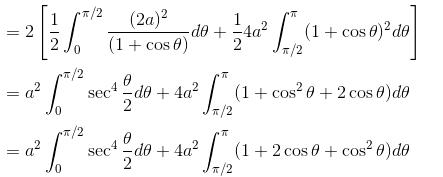By putting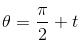in the second integral and by putting θ/2 =  u in the first integral